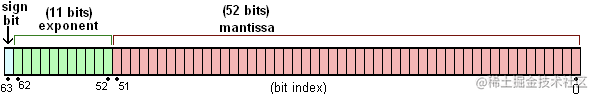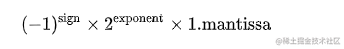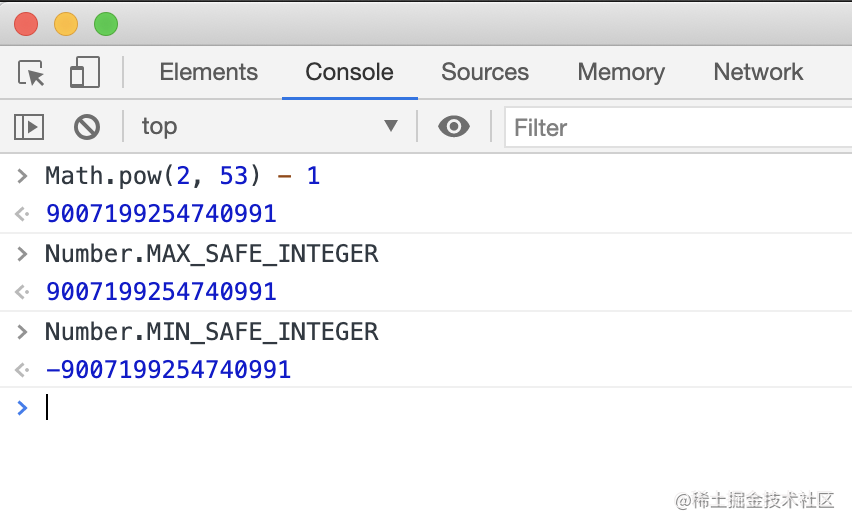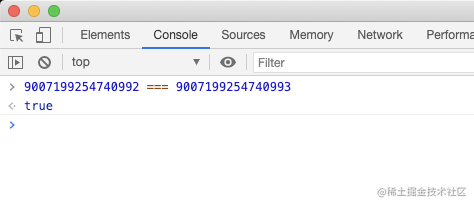# 硬核基础二进制篇（一）0.1 + 0.2 != 0.3 和 IEEE-754 标准

2021-08-18 3:17:4420

## Javascript 是怎么存储数字的 —— IEEE-754 标准

JavaScript 的数字是 IEEE-754 标准存储的双精度浮点数类型。双精度浮点数总共有 64 位（bit），第一位用于表示符号，接着十一位用于表示阶码，剩余的五十二位用于表示尾数。### 阶码（exponent）

``````01111111111 // 0
10000000000 // 1
11111111110 // 1023
00000000000 // -1024
``````

### 尾数 (mantissa)

``````(0.625)10 = 0 + 6 * 10^-1 + 2 * 10^-2 + 5*10^-3
``````

``````(0.101)2 => 0 + 2^-1 + 2^-3 => 十进制的 1/2 + 1/8 = 0.625
``````

``````0 00000000000 0000000000000000000000000000000000000000000000000000
``````

### Number.MAX_SAFE_INTEGER 是怎么来的

`111...111(53 个 1)` = `1000...000(53 个 0) - 1` 也就是 `2^53 - 1``9007199254740992` 的二进制形式为：

``````0 10000110100 0000000000000000000000000000000000000000000000000000
``````

`9007199254740993` 的二进制形式也是！！！

``````0 10000110100 0000000000000000000000000000000000000000000000000000
``````### 0.1 + 0.2 !== 0.3

``````15 % 2 === 1, 15 => 7
7 % 2 === 1, 15 => 3
3 % 2 === 1, 3 => 1
1 % 2 === 1, 1 => 0
``````

``````0.125 * 2 => 0.25, 0
0.25 * 2 => 0.5, 0
0.5 * 2 => 1, 1
``````

``````0.1 * 2 => 0.2, 0
0.2 * 2 => 0.4, 0
0.4 * 2 => 0.8, 0
0.8 * 2 => 1.6, 1
0.6 * 2 => 1.2, 1
0.2 * 2 => 0.4, 0
...
``````

``````0 01111111011 1001100110011001100110011001100110011001100110011010
``````

`0.2``0.1` 的两倍，尾码保持不动，阶码 + 1，得到 `0.2` 的二进制形式：

``````0 01111111100 1001100110011001100110011001100110011001100110011010
``````

0.2 的阶码比 0.1 的阶码大一，我们把 0.1 的尾码右移一位，阶码减 1，让两个数的阶码保持一致

``````0 01111111100 0.1100110011001100110011001100110011001100110011001101
0 01111111100 1.1001100110011001100110011001100110011001100110011010
``````

``````  0 01111111100  0.1100110011001100110011001100110011001100110011001101
+ 0 01111111100  1.1001100110011001100110011001100110011001100110011010
= 0 01111111100 10.0110011001100110011001100110011001100110011001100111
``````

``````0 01111111101 0011001100110011001100110011001100110011001100110011 1（1 多出，需要舍弃）
0 01111111101 0011001100110011001100110011001100110011001100110100  （补 1）
``````

``````0 01111111101 0011001100110011001100110011001100110011001100110100
``````

``````0.3 * 2 => 0.6, 0
0.6 * 2 => 1.2, 1
0.2 * 2 => 0.4, 0
0.4 * 2 => 0.8, 0
0.8 * 2 => 1.6, 1
0.6 * 2 => 1.2, 1
...
``````

``````0 01111111101 0011001100110011001100110011001100110011001100110011
``````

`0.1 + 0.2` 的运算结果确实不相等，至此我们总算搞明白了，在浮点数运算过程中的误差问题。总结一下就是，小数在计算机的存储过程中本身就存在精度丢失的问题，然后尾数的位数总共只有 `52` 位，放不下时会被丢弃，并按照 `舍0补1` 来弥补导致最终运算结果不相等。

## 相关链接苏公网安备 32030302000848号   苏ICP备20033101号-1